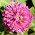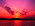Tuesday, January 13, 2009

A Number Game by Will ShortzThe computer lady gave me this puzzle today and I can't do a thing until I solve all of the equations. This is the text that accompanied the puzzle:
This test gauges your mental flexibility. Few have been found who could solve more than half of the questions on the first try. The puzzle originally consisted of 24 "equations" by Will Shortz, printed in the May-June 1981 issue of Games Magazine [to which I subscribe!], with an acknowledgement to Morgan Worthy.

Instructions: Each equation contains the initials of words that will make it correct. furnnish the missing words. (example: 60 = M in an H. Answer: 60 = Minutes in an Hour.) Good luck!
 1 = W on an U2 = Number it T to T3 = B M (S H T R)4 = Q in a G4 = Q in a G (Note: this Q in aG is a different answer thanthe previous one.)5 = D in a Z C6 = L on an I7 = W of the A W8 = S on a S S9 = P in the S S9 = L a C has10 = D in a T N with the A C11 = P on a F T12 = K of the R T13 = S on the A F18 = H in a G C20 = F and T21 = D on a D 26 = L of the A29 = D in F in a L Y32 = D F at which W F40 = D and N of the G F52 = W in a Y52 = C in a D54 = C in a D (with the J)57 = H V64 = S on a C80 = D to G A the W88 = P K90 = D in a R A99 = B of B O the W200 = D for P G in M1000 = W that a P is W1001 = A N2001 = a S O20,000 = L U the S

I have solved some of these and I have found a spoiler (which I am not using!). My solutions and the spoiler URL are in the comments. Add your own solutions!

Technorati Tags:

_/\_/\_

1.Hey there: the spoiler is at
http://www.greenleecds.com/rgbest/NumAKey.pdf

The ones I have solved today:

20=Fingers and Toes
29=Days in February in a Leap Year
40=Days and Nights of the Great Flood
52=Days in a Year
99=Bottles of Beer On the Wall
1001=Arabian Nights
20,000=Leagues Under the Sea

Have any more solutions?

2.I love this guy on pbs radio on Sunday.

Coffee is on.

3.I could solve some:
4 = quarts in a gallon
4 = quarters in a game
6 = legs on an insect
7 = wonders of the ancient world
9 = lives a cat has
11 = players on a football team
12 = Knights of the Round Table
18 = holes in a golf course
26 = letters of the alphabet
32 = degrees Fahrenheit at which water freezes
52 = cards in a deck
54 = cards in a deck with the Joker
57 = Heinz varieties
64 = squares on a chessboard
80 = days to go around the world
90 = degrees in a right angle

Still at a loss for the others.

4.How about creating a few new ones?
Such as 1 = P in a P T
7 = S a S
12 = L a L

...........any guesses????

(Hi, A. I haven't forgotten all I owe you, literally and figuratively. I haven't been able to be around... but things may be improving....FINALLY.....)

Thank you for visiting and for your comments!